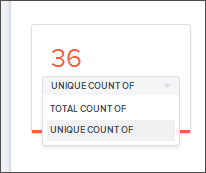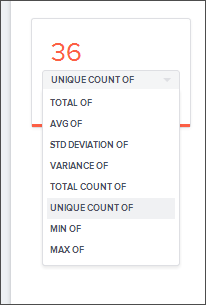Aggregation is grouping many units or parts into a new value. In data, aggregation gathers multiple input values and calculates a summary value from them. You then use this aggregated value to do an analysis.

Every summary value results from a data aggregation function. An example aggregation function would be average or minimum. You can control how aggregation works in your data.

## Making an ATTRIBUTE column ADDITIVE

Your data may contain a column with a numeric data type that you have defined as an `ATTRIBUTE` rather than a `MEASURE`. For example, you may have `ATTRIBUTE` column with an `INTEGER` data type that represents age. Typically, these columns have an ADDITIVE setting of `NO`. Within a search result that contains data from this column, the options for each column on the left side of the screen includes:

• UNIQUE COUNT OF

• TOTAL COUNT OFTo display extended aggregate view options, you must set ADDITIVE to `YES` on these `ATTRIBUTE` columns. This option is only possible on columns that have a numeric data type (`FLOAT`, `DOUBLE` or `INTEGER`) or a date data type (`DATE`, `DATETIME`, `TIMESTAMP`, or `TIME`). After you make this change, these additional view options area-charts offered:

• TOTAL OF

• AVG OF

• STD DEVIATION OF

• VARIANCE OF

• TOTAL COUNT OF

• UNIQUE COUNT OF

• MIN OF

• MAX OFTo change this setting:

1. Find the column whose ADDITIVE setting you want to change

3. Change the value to one of these:

• `YES` or `NO`, if using the Web interface.

• `TRUE` or `FALSE`, if using the model file.

## Change Aggregation

Both `MEASURE` columns and `ATTRIBUTE` columns support AGGREGATION operations. To aggregate a column without having to enter the aggregation type explicitly in your searches every time, you can set a default Aggregation for that column. Setting this default can make combining data more intuitive and faster.

`ATTRIBUTE` columns have AGGREGATION(UI)/AggregationType (model file) values with default aggregate type of NONE. You can change AGGREGATION to one of the supported aggregation types. To extend the available aggregation actions, set ADDITIVE on these columns to `YES` (`TRUE`).

Aggregate type Description

NONE

Does no aggregation. This is the default for `ATTRIBUTE` type columns.

SUM

Adds the values together and returns the total. This is the default for `MEASURE` type columns.

AVERAGE

Calculates the average of all the values.

MIN

Calculates the minimum value.

MAX

Calculates the maximum value.

STD_DEVIATION

Calculates the standard deviation of all the values.

VARIANCE

Calculates the variance of all the values.

COUNT

Calculates the total number of values.

COUNT_DISTINCT

Calculates the total number of distinct values.

Keep in mind that not all numeric data should be aggregated. Consider a table containing data about athletes on a sports team. The data contains some numerical values, including points scored, salaries, and jersey numbers for each of the players. You should aggregate points scored and salaries, but it is not meaningful to aggregate jersey numbers. Because jersey number is an INTEGER, it would become a column of type `MEASURE` (not `ATTRIBUTE`), so it will aggregate, by default. You must manually change it to a column of type `ATTRIBUTE`. This ensures that search results that include jersey number will not attempt to compare or aggregate those values in a way that is not meaningful.

To set the aggregation value, follow these steps:

1. Find the column whose default aggregation type you want to change

2. Select its Aggregation. If using the modeling file, use the AggregationType setting.

3. Select the new default aggregation type.